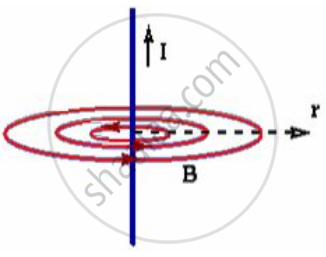# State Ampere’S Circuital Law. - Physics

State Ampere’s circuital law.

#### Solution 1

Statement: The line integral of the magnetic field (vecB) around any closed path is equal to μtimes
the total current (I) passing through that closed path.

:.ointvecB.vec(dl)=mu_0I

#### Solution 2

Statement: The line integral of the magnetic field (vecB) around any closed path is equal to μtimes
the total current (I) passing through that closed path.

:.ointvecB.vec(dl)=mu_0I

#### Solution 3

Ampere’s law states that the path integral or line integral over a closed loop of the magnetic field produced by a current distribution is given by oint vec("B") . vec("dl") = µ_0"l"

where I refers to the current enclosed by the loop.

Ampere’s law is a useful relation that is analogous to Gauss’s law of electrostatics. It is a relation between the tangential component of magnetic field at points on a closed curve and the net current through the area bounded by the curve.

To evaluate the expression for oint vec("B") . vec("dl") let us consider a long, straight conductor carrying a current I, passing through the centre of a circle of radius r in a plane perpendicular to the conductor.According to Biot-Savart law of magnetism, the field has a magnitude (µ_0"l")/(2pir) at every point on the circle, and it is tangent to the circle at each point.

The line integral of vec("B") around the circle is

oint vec("B") . vec("dl") = oint(µ_0"I")/(2pir)"dl" = (µ_0"l")/(2pir) oint"dl"

Since ointvec("dI") = 2pir is the circumference of the circle,

Therefore , oint vec("B") . vec("dl") = µ_0"l"

Concept: Ampere’s Circuital Law
Is there an error in this question or solution?
2013-2014 (March)

Share